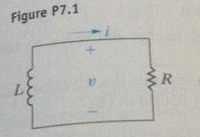# Problem: In the Circuit in Fig. p7.1, the voltage and current expressions are:v = 160e–10t V, t &gt; =0+;i = 6.4e–10t A, t &gt; = 0.Finda) R.b) τ ( in milliseconds).c) L.d) the initial energy stored in the inductor.e) the time (in milliseconds) it takes to dissipate 60% of the initial stored energy.

###### FREE Expert Solution

In this problem, we're going to use Ohm's law.

Ohm's law:

$\overline{){\mathbit{i}}{\mathbf{=}}\frac{\mathbf{V}}{\mathbf{R}}}$, where i is current, V is voltage, and is resistance.

a)

From Ohm's law, we can get resistance as:

R = V/i

Substituting the expressions for V and i that we've been given:

96% (471 ratings)###### Problem Details

In the Circuit in Fig. p7.1, the voltage and current expressions are:

v = 160e–10t V, t > =0+;

i = 6.4e–10t A, t > = 0.Find

a) R.

b) τ ( in milliseconds).

c) L.

d) the initial energy stored in the inductor.

e) the time (in milliseconds) it takes to dissipate 60% of the initial stored energy.